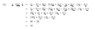# Dot product of vector and a dyadic.

htdIO
Question
If a = i - 2j + 2k
b = 4i - 3j
M = 3ii + 2ij - ji + kj. M is a dyadic.
Determine a$\bullet$2M$\bullet$b

## The Attempt at a Solution

This isn't really homework, but rather just some self study to help me understand some of my work better. Attached is the answer.

My real question in this case is some info on how the dot product works? Been trying to find some info about this for 2 days now. If someone could just help explain the dot product between a and 2M, I would be really greatful.

Thanks

#### Attachments

•7.9 KB · Views: 1,283

voko
If that is self-study, then surely the book(s) you use should explain the dot product before giving you examples of this kind? What exactly do you not understand in your books?

htdIO
No, the book we use makes the assumption that we know how to calculate the dot product and only introduces dyadics.

I know how to calculate the dot product of 2 vectors if they were something like this:

(4i + 2j) (dot) (i + j) = 4+2 = 6.

But those vectors are of the same size. How do you go about it in the example, where the vector sizes differ?

Homework Helper
One vector is 4i+2j and the other is i+j right? Then those two vectors are not the same size. (And when I say 'size', I mean inner product).

Staff Emeritus
Homework Helper
When you multiply 2M on the left by a, you deal with the unit vectors on the left in each term of the dyad. Similarly, when you multiply on the right by b, you deal with the unit vectors on the right. That's all there is to it.

voko
No, the book we use makes the assumption that we know how to calculate the dot product and only introduces dyadics.

I know how to calculate the dot product of 2 vectors if they were something like this:

(4i + 2j) (dot) (i + j) = 4+2 = 6.

But those vectors are of the same size. How do you go about it in the example, where the vector sizes differ?

It is a rather strange book that you have. If it introduces something, it should explain how that something works. For a dyad $\textbf{ab}$ and a vector $\textbf{c}$: $$\textbf{c} \cdot \textbf{ab} = (\textbf{c} \cdot \textbf{a})\textbf{b}$$$$\textbf{ab} \cdot \textbf{c}= \textbf{a}(\textbf{b} \cdot \textbf{c})$$

htdIO
It is a bit frustrating.

Thanks for all the answers, I've realized my mistake.

I somehow messed forgot the right definition for dot product. Just grabbed some old books and everything seems to work out now.

Sorry and thanks again for all the help.

$$\begin{bmatrix}6 & 4 & 0 \\ -2 & 0 & 0 \\ 0 & 4 & 0\end{bmatrix}$$
$$\begin{bmatrix}1 & -2 & 2\end{bmatrix}\begin{bmatrix}6 & 4 & 0 \\ -2 & 0 & 0 \\ 0 & 4 & 0\end{bmatrix}\begin{bmatrix}4\\ -3 \\ 0\end{bmatrix}$$